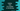# Python Program to find if a number is Armstrong or not## Find if a number is Armstrong or not using python :

A three-digit number is called an Armstrong number if the sum of cube of its digits is equal to the number itself. For example, 407 is an Armstrong number since`4****3 + 0****3 + 7****3 = 64 + 0 + 343 = 407`. As you can see, the sum of the cubes of its digits is equal to the number. Note that not all Armstrong numbers are of three digits. We can have Armstrong numbers with any number of digits. For a number with ‘n’ digits, the power value should be n, not 3. i.e. for a 4 digit number 1234, we need to check the value of `1****4 + 2****4 + 3****4 + 4**4` to determine if it is an Armstrong number or not. In this tutorial, we will learn how to find out if a number is Armstrong or not using python. The program will ask the user to enter a number. It will read that number and print out the result to the user.

## Python program to check if a number is Armstrong or not :

``````def findArmStrongSum(no):
currentNo = no
length = len(str(currentNo))
sum = 0

while currentNo > 0:
lastDigit = currentNo % 10
sum += lastDigit ** length

currentNo = int(currentNo/10)

return sum

no = int(input("Enter a positive number :"))

if(no>0):
armStrongSum = findArmStrongSum(no)
if(armStrongSum == no):
print ("Given number is an Armstrong Number")
else:
print ("Number is not an Armstrong Number”)
else:
print ("Please enter a valid number")``````

### Explanation :

1. In the example above, we have defined one method findArmStrongSum to find out the required sum for a number. This method takes one number as its parameter and returns the sum. Note that this program will work with any digit numbers. As explained above, for a number with ‘n’ digits, the power value will be ‘n’. e.g. for ‘407’, the power value will be ‘3’ and for ‘23’, the power value will be ‘2’. To find out the power value, we are calculating the total digits in the given number. The str() method converts one number to a string and if we wrap it with len(), we can have the total count of digits.
2. The total count of digits is stored in the ‘length’ variable. One variable ‘sum’ is initialized as ‘0’ to store the required sum.
3. The while loop is used to find out the sum of all powers. It calculates the sum recursively. If you are getting confused here, try to run the program by printing out the variables used inside the loop. It will make everything more transparent to you.
4. Using the input() method, ask the user to enter a positive number. This is the line that actually executes at the start of the program. input() method returns the value as a string. We are converting this value to an integer by wrapping with int() method. This value is stored in the ‘no’ variable.
5. Next, we are checking again if the user input number is more than 0 or not. If yes, we are finding the total sum using the ‘findArmStrongSum’ method defined above. This value is stored in the ‘armStrongSum’ variable. If the number is not greater than 0, we are printing one message asking the user to enter a valid number.
6. Finally, we are comparing the sum with the given number and we are printing one message accordingly.

## Find out all Armstrong Numbers in a range :

We can also find out all Armstrong numbers in a range easily using a loop. Let’s find out all Armstrong numbers within range 0 to 1000 :

### Python Program :

``````def findArmStrongSum(no):
currentNo = no
length = len(str(currentNo))
sum = 0

while currentNo > 0:
lastDigit = currentNo % 10
sum += lastDigit ** length

currentNo = int(currentNo/10)

return sum

strongNumList = []

for i in range(0,1000):
armStrongSum = findArmStrongSum(i)
if (armStrongSum == i):
strongNumList.append(i)

for no in strongNumList :
print (no)``````